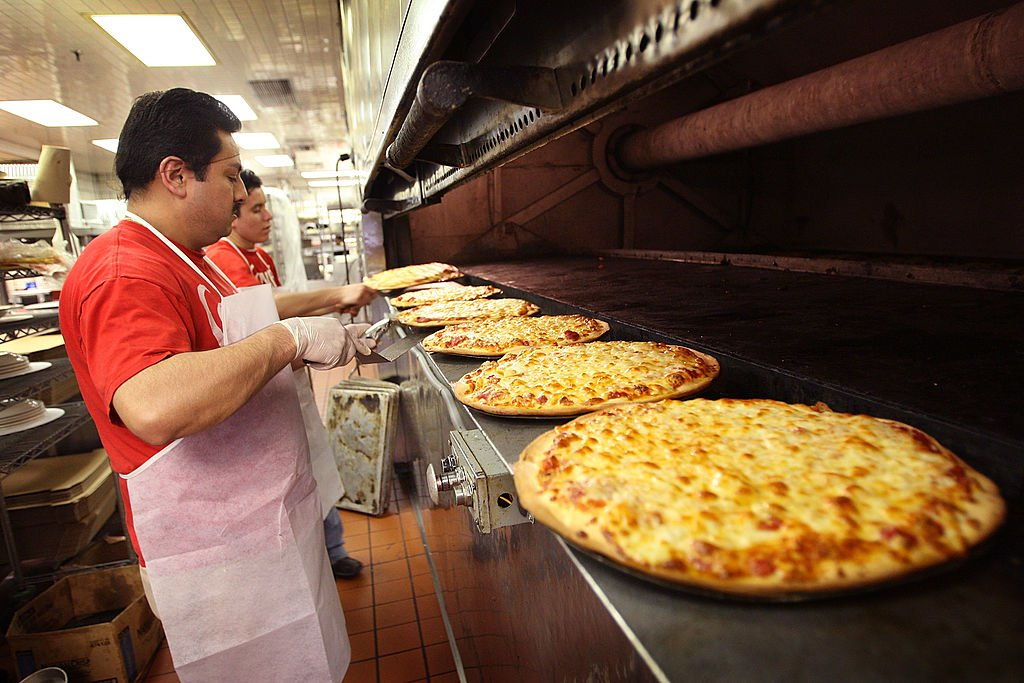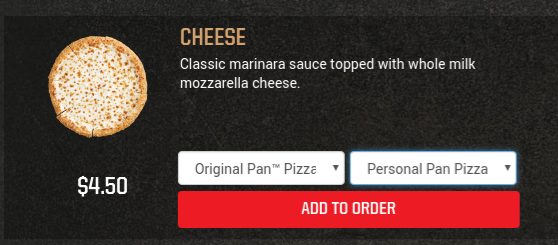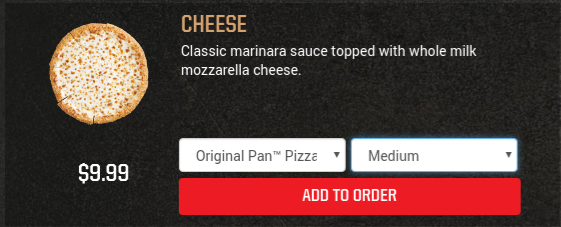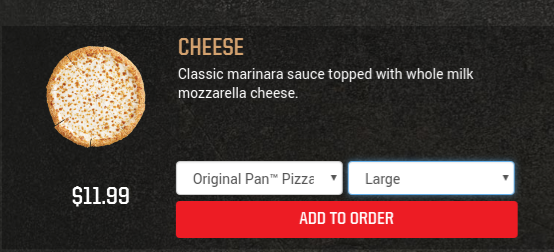Surprising Science

# The Science Is In: You Should Always Order the Biggest Pizza

A new study broke down the pizza prices from 3,678 pizza joints throughout the United States to see if ordering a large pizza is the best deal. It is. We break down the price per square inch of pizza from three options at Pizza Hut.

Quick: how much bigger is a 16-inch pizza than an 8-inch pizza?

If you answered twice the size, we may need to send you in for some remedial math. A 16-inch pizza is four times the size of the 8-inch pizza. Size matters. An extensive study by NPR examined the prices from 3,678 pizza joints throughout the United States (with data from Grubhub Seamless), which calculated the price per square inch of pizza, came to this conclusion:

You should always order the large pizza. It will be less per square inch of pizza.

While a 16-inch pizza is four times the size of the 8-inch pizza, the larger pizza will be far less than four times the price of the small. Although we obviously expect a discount per unit (square inch for pizza in this case), our difficulty with quickly understanding the size difference may make the price differences seem less extreme than it truly is. [I break down the prices at Pizza Hut later in this article.]

Judging the value we get per unit is not always clear just from our eyes–that’s why grocery stores typically provide a price-per-unit for consumers.The Size of a Pizza Pie

In order to figure out the size of a pizza pie, you literally have to use π (pi) in the calculation. If it has been awhile since you sat in an uncomfortable seat with a #2 pencil, π is equal to approximately 3.14159. The calculation for the area of the pizza is πr2. R, the radius, is half the diameter.

16-inch pizza:

Calculation: 3.14159 * (8 squared) = 201.06176

8-inch pizza:

Calculation: 3.14159 * (4 squared) = 50.26544The Price of Pizza at Pizza Hut

While the NPR study looked at over 3,000 pizza places to crunch the average price per square inch of pizza, it can be helpful to look at just one popular eatery–Pizza Hut. Pizza Hut serves three sizes: personal pan, medium, and large. Note: eateries do not always clearly list the size of their pizza in inches.[A personal pan at Pizza Hut is 6 inches in diameter.]

Size Calculation: 3.14159 * (3 squared) = 28.27431

Price per square inch of pizza = \$4.50/28.27431 = 15.92 cents per square inch[A medium pizza at Pizza Hut is 12 inches in diameter.]

Size Calculation: 3.14159 * (6 squared) = 113.09724

Price per square inch of pizza = \$9.99/113.09724 = 8.83 cents per square inch of pizza[A large pizza at Pizza Hut is 14 inches in diameter.]

Size Calculation: 3.14159 * (7 squared) = 153.93791

Price per square inch of pizza = \$11.99/153.93791 = 7.79 cents per square inch of pizza

Pizza + Math = Delicious dealRelated

Up Next